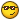## Recommended Posts

Hey Guys,

Looking for any help you can give me on this one. I need to get a script together that will do the following:

a) Prompt to browse for the default spreadsheetPrompt to determine how many rows should be read

c) Read each row of data starting in cell A9 to M9 and down the number of rows in step (d) Transfer each row of data to a text file with each cells data separated by commas.

The prompts and stuff are easy obviously it's reading the data from Excel and transfering it to the text file I can figure out. Any help would be greatly appreciated.

Thanks,

Michael

Edited by ca143508

##### Share on other sites

May be this might help you...Also, I do not know who originally wrote this code.

You will probably be interested in this part of the code...

\$Values = ""

For \$c In \$NewBook.Sheets("Sheet1").Range("a1:n9").Cells

\$Values = \$Values & \$c.Text & @TAB

Next

```\$Excel = ObjCreate("Excel.Application"); Create an Excel Object
if @error then
Msgbox (0,"","Error creating Excel object. Error code: " & @error)
exit
endif
if not IsObj(\$Excel) then
Msgbox (0,"ExcelTest","I'm sorry, but creation of an Excel object failed.")
exit
endif
\$Excel.Visible = 1  ; Let the guy show himself - Set to 0 to run excel invisible
; Example: Fast Fill some cells with there address
Msgbox (0,"","Click 'ok' to fastfill some cells")
For \$c In \$NewBook.Sheets("Sheet1").Range("a1:n1").Cells
For \$i = 1 To 8
Next
Next

msgbox(0,"ExcelTest","Click 'ok' to read the cells in each column.")
\$Values = ""
For \$c In \$NewBook.Sheets("Sheet1").Range("a1:n9").Cells
\$Values = \$Values & \$c.Text & @TAB
Next
\$Excel.activeworkbook.saved = 1; To prevent 'yes/no' questions from Excel
\$Excel.quit       ; Get rid of him.
\$Excel = 0        ; Loose this object.
; Object will also be automatically discarded when you exit the script
exit```

##### Share on other sites

May be this might help you...Also, I do not know who originally wrote this code.

You will probably be interested in this part of the code...

\$Values = ""

For \$c In \$NewBook.Sheets("Sheet1").Range("a1:n9").Cells

\$Values = \$Values & \$c.Text & @TAB

Next

```\$Excel = ObjCreate("Excel.Application"); Create an Excel Object
if @error then
Msgbox (0,"","Error creating Excel object. Error code: " & @error)
exit
endif
if not IsObj(\$Excel) then
Msgbox (0,"ExcelTest","I'm sorry, but creation of an Excel object failed.")
exit
endif
\$Excel.Visible = 1; Let the guy show himself - Set to 0 to run excel invisible
; Example: Fast Fill some cells with there address
Msgbox (0,"","Click 'ok' to fastfill some cells")
For \$c In \$NewBook.Sheets("Sheet1").Range("a1:n1").Cells
For \$i = 1 To 8
Next
Next

msgbox(0,"ExcelTest","Click 'ok' to read the cells in each column.")
\$Values = ""
For \$c In \$NewBook.Sheets("Sheet1").Range("a1:n9").Cells
\$Values = \$Values & \$c.Text & @TAB
Next
\$Excel.activeworkbook.saved = 1; To prevent 'yes/no' questions from Excel
\$Excel.quit     ; Get rid of him.
\$Excel = 0      ; Loose this object.
; Object will also be automatically discarded when you exit the script
exit```

Thanks for thi code PR. I have made some changes to get it pretty close to the mark but am struggling with one last item. When it hits the end of the row, I need the file write line to get an @CRLF command. Any ideas??? I have added the | so that should we need to put the data back into a dbase or excel it can be identified as delimited.

\$file = FileOpenDialog("","C:\","Microsoft Excel (*.xls)",1)

\$exceldoc = ObjGet(\$file)

\$Values = ""

For \$c In \$exceldoc.Sheets("Sheet1").Range("a8:t150").Cells

\$Values = \$Values & \$c.Text & "|"

Next

FileWriteLine("C:\Test.txt",StringStripWS(\$Values,7) & @CRLF)

exit

Cheers,

Michael

Edited by ca143508

##### Share on other sites

Well it's hard to say without seeing all of th code...So I'm assuming that the range of cells you where interested in had been sent to the file Test.txt.

You can try something like this...

\$file = FileOpen("c:\test.txt", 2)

....................................

....................................

FileWriteLine(\$file,StringStripWS(\$Values,7) & @CRLF)

Exit

FileWriteLine(\$file," " & @CRLF)

FileClose(\$file)

##### Share on other sites

Well it's hard to say without seeing all of th code...So I'm assuming that the range of cells you where interested in had been sent to the file Test.txt.

You can try something like this...

\$file = FileOpen("c:\test.txt", 2)

....................................

....................................

FileWriteLine(\$file,StringStripWS(\$Values,7) & @CRLF)

Exit

FileWriteLine(\$file," " & @CRLF)

FileClose(\$file)

What I have added to my previous post is all the code I was using to dump the contents into a text file. The problem is it is writing it in all one long line. I want each row of the original spreadsheet to go on it's own line in the text file. So ie/ a9:t9 on the first line. a10:t10 on the second and so on. Does that make sense???

Cheers,

Michael

Edited by ca143508

##### Share on other sites

This should do the trick...

```\$Excel = ObjCreate("Excel.Application"); Create an Excel Object
if @error then
Msgbox (0,"","Error creating Excel object. Error code: " & @error)
exit
endif
if not IsObj(\$Excel) then
Msgbox (0,"ExcelTest","I'm sorry, but creation of an Excel object failed.")
exit
endif
\$Excel.Visible = 1; Let the guy show himself - Set to 0 to run excel invisible
; Example: Fast Fill some cells with there address
Msgbox (0,"","Click 'ok' to fastfill some cells")
For \$c In \$NewBook.Sheets("Sheet1").Range("a1:n1").Cells
For \$i = 1 To 8
Next
Next
\$TextFile = "C:\TextFile.txt"
FileOpen(\$TextFile, 2)
msgbox(0,"Excel2Text","Click 'ok' to copy cells into a single column text file")
\$Values = ""
For \$c In \$NewBook.Sheets("Sheet1").Range("a1:n9").Cells
\$Values = \$c.Text & @TAB
FileWriteLine(\$TextFile, \$Values)
Next
\$Excel.activeworkbook.saved = 1; To prevent 'yes/no' questions from Excel
\$Excel.quit    ; Get rid of him.
\$Excel = 0     ; Loose this object.
; Object will also be automatically discarded when you exit the script

FileClose(\$TextFile)
exit```

##### Share on other sites

Thanks for this. I'll give it a try and see how it goes.

Michael.

## Create an account

Register a new account

×

• Wiki

• Back

• #### Beta

• Git
• FAQ
• Our Picks
×
• Create New...